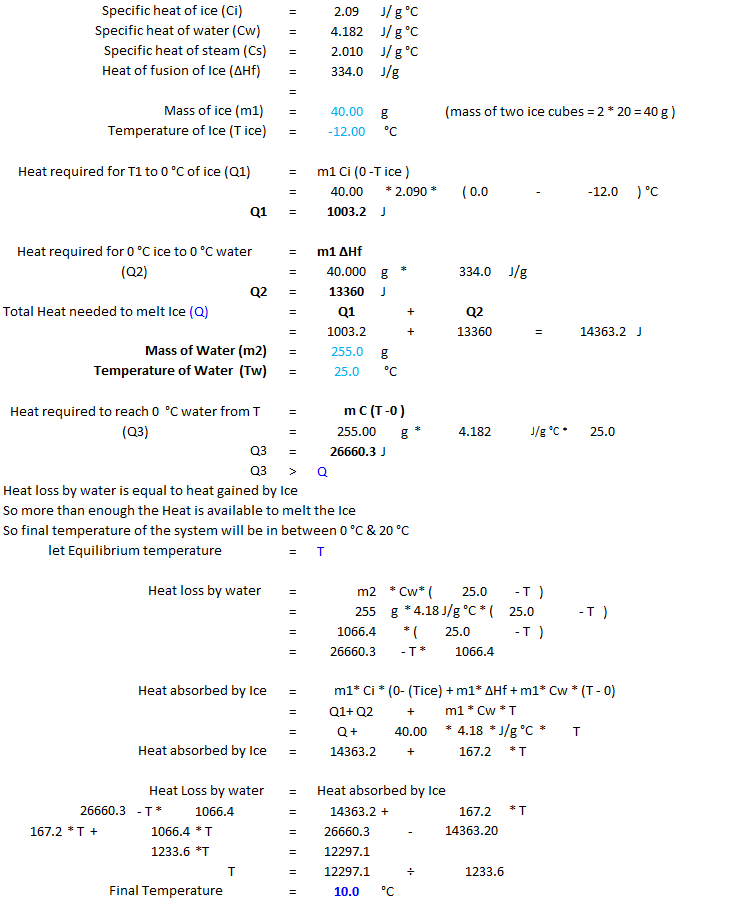In: Chemistry

# Two 20.0 g ice cubes at −12.0 ∘C are placed into 255 gof water at 25.0...

Two 20.0 g ice cubes at −12.0 ∘C are placed into 255 gof water at 25.0 ∘C. Assuming no energy is transferred to or from the surroundings, calculate the final temperature, Tf, of the water after all the ice melts.

## Solutions

##### Expert Solution## Related Solutions

##### Two 20.0-g ice cubes at –20.0 °C are placed into 225 g of water at 25.0...
Two 20.0-g ice cubes at –20.0 °C are placed into 225 g of water at 25.0 °C. Assuming no energy is transferred to or from the surroundings, calculate the final temperature of the water after all the ice melts.
##### Two 20.0-g ice cubes at –21.0 °C are placed into 295 g of water at 25.0...
Two 20.0-g ice cubes at –21.0 °C are placed into 295 g of water at 25.0 °C. Assuming no energy is transferred to or from the surroundings, calculate the final temperature of the water after all the ice melts. heat capacity of H2O(s) 37.7 heat capacity of H2O (l) 75.3 enthalpy of fusion of H2O 6.01
##### Two 20.0-g ice cubes at –19.0 °C are placed into 245 g of water at 25.0...
Two 20.0-g ice cubes at –19.0 °C are placed into 245 g of water at 25.0 °C. Assuming no energy is transferred to or from the surroundings, calculate the final temperature, Tf, of the water after all the ice melts. Heat capacity of H20(s) = 37.7J/(mol*K) Heat cap of H20(l) 75.3 J/(mol*K) enthalpy of fusion of H20 = 6.01 kJ/mol
##### Two 20.0-g ice cubes at –16.0 °C are placed into 275 g of water at 25.0...
Two 20.0-g ice cubes at –16.0 °C are placed into 275 g of water at 25.0 °C. Assuming no energy is transferred to or from the surroundings, calculate the final temperature of the water after all the ice melts. Given the Following Values: Heat Capacity of H2O (s) = 37.7 J/mol*K Heat Capacity of H2O (l) = 75.3 J/mol*K Enthalpy of Fusion of H2O = 6.01 kJ/mol
##### Two 20.0-g ice cubes at –19.0 °C are placed into 215 g of water at 25.0...
Two 20.0-g ice cubes at –19.0 °C are placed into 215 g of water at 25.0 °C. Assuming no energy is transferred to or from the surroundings, calculate the final temperature of the water after all the ice melts.
##### Two 20.0-g ice cubes at –21.0 °C are placed into 225 g of water at 25.0...
Two 20.0-g ice cubes at –21.0 °C are placed into 225 g of water at 25.0 °C. Assuming no energy is transferred to or from the surroundings, calculate the final temperature of the water after all the ice melts.
##### Two 20.0 g ice cubes at −11.0 ∘C are placed into 225 g of water at...
Two 20.0 g ice cubes at −11.0 ∘C are placed into 225 g of water at 25.0 ∘C. Assuming no energy is transferred to or from the surroundings, calculate the final temperature, Tf, of the water after all the ice melts. heat capacity of H2O(s)H2O(s) 37.7 J/(mol⋅K) heat capacity of H2O(l)H2O(l) 75.3 J/(mol⋅K) enthalpy of fusion of H2OH2O 6.01 kJ/mol Tf= ∘C
##### two 20g ice cubes at -21 c are placed into 215g of water at 25 c...
two 20g ice cubes at -21 c are placed into 215g of water at 25 c assuming no energy is transfered to or from the surroundings, calculate the final temperature of the water after all the ice melts.
##### A sheet of gold weighing 12.0 g and at a temperature of 20.0°C is placed flat...
A sheet of gold weighing 12.0 g and at a temperature of 20.0°C is placed flat on a sheet of iron weighing 21.5 g and at a temperature of 59.4°C. What is the final temperature of the combined metals? Assume that no heat is lost to the surroundings. A 34.51−g stainless steel ball bearing at 104.89 °C is placed in a constant-pressure calorimeter containing 116.9 g of water at 22.47°C. If the specific heat of the ball bearing is 0.474...
##### (a) Two 29 g ice cubes are dropped into 170 g of water in a thermally...
(a) Two 29 g ice cubes are dropped into 170 g of water in a thermally insulated container. If the water is initially at 26°C, and the ice comes directly from a freezer at -16°C, what is the final temperature at thermal equilibrium? (b) What is the final temperature if only one ice cube is used? The specific heat of water is 4186 J/kg·K. The specific heat of ice is 2220 J/kg·K. The latent heat of fusion is 333 kJ/kg.